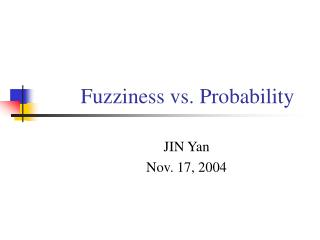DownloadDownload PresentationFuzziness vs. Probability

# Fuzziness vs. Probability

Télécharger la présentation## Fuzziness vs. Probability

- - - - - - - - - - - - - - - - - - - - - - - - - - - E N D - - - - - - - - - - - - - - - - - - - - - - - - - - -
##### Presentation Transcript

1. Fuzziness vs. Probability JIN Yan Nov. 17, 2004

2. The outline of Chapter 7 Part I Fuzziness vs. probability Part II Fuzzy sets & relevant theories

3. Part I In general, Kosko's position is: • Probability is a subset of Fuzzy Logic. • Probability still works. It is just the binary subset of fuzzy theory.

4. Fuzziness in a Probabilistic World Uncertainty—how to describe this notion in math? • Probability is the only answer ( probabilists hold this.) • Fuzziness. (probability describes randomness, but randomness doesn’t equal uncertainty. Fuzziness can describe those ambiguous phenomena which are also uncertainty.)

5. Randomness vs. Fuzziness • Similarities: 1. both describe uncertainty. 2. both using the same unit interval [0,1]. 3. both combine sets & propositions associatively, commutatively, distributively.

6. Randomness vs. Fuzziness • Distinctions: • Fuzziness  event ambiguity. Randomness  whether an event occurs. • How to treat a set A & its opposite Ac ( key one). • Probability is an objective characteristic Fuzziness is subjective. ( The last viewpoint comes from “Fuzziness versus probability again”, F. Jurkovič )

7. Randomness vs. Fuzziness • The 1st distinction and the 3rd one: Example1: • There is a 20% chance to rain. ( probability & objectiveness ) • It’s a light rain. ( fuzziness & subjectiveness) Example 2: • Next figure will be an ellipse or a circle, a 50% chance for every occasion. ( probability & objectiveness ) • Next figure will be an inexact ellipse. ( fuzziness & subjectiveness)

8. or An inexact ellipse

9. Randomness vs. Fuzziness • The key distinction: Is always true ? The formula describes the law of excluded middle --- white or black. Yet how about those “a bit white & a bit black” events? ---- Fuzziness!!

10. Part II • Fuzzy sets & relevant theories: • the geometry of fuzzy sets (√) • the fuzzy entropy theorem • the subsethood theorem

11. the Geometry of Fuzzy Sets: Sets as Points • Membership function maps the domain X = {x1, ……, xn} to the range [0, 1]. That is X  [0, 1]. • The sets-as-points theory: the fuzzy power set F(2X) In [0, 1]n (unit hypercube) a fuzzy set  a point in the cube In nonfuzzy set  the vertices of the cube midpoint  maximally fuzzy point (if , A is the midpoint)

12. the Geometry of Fuzzy Sets: Sets as Points Sets as points.The fuzzy subset A is a point in the unit 2-cube with coordinates (1/3, 3/4). The 1st element x1(2nd, x2)belongs to A to degree 1/3(3/4).

13. the Geometry of Fuzzy Sets: Sets as Points • Combine set elements with the operators of Lukasiewicz continuous logic: Proposition: A is properly fuzzy iff , iff

14. the Geometry of Fuzzy Sets: Sets as Points The fuzzier A is, the closer A is to the midpoint (black hole ) of the fuzzy cube. The less fuzzy A is, the closer A is to the nearest vertex.

15. the Geometry of Fuzzy Sets: Sets as Points • The midpoint is full of paradox!! Bivalent paradox examples: e.g.1A bewhiskered barber states that he shaves a man iff he doesn’t shave himself. a very interesting question rise: who shaves the barber? let S the proposition that the barber shaves himself, not-S  he does not, then the S & not-S are logically equivalent t( S ) = t (not-S ) = 1 - t( S )  t( S ) = 1/2

16. the Geometry of Fuzzy Sets: Sets as Points e.g. 2 One man said: I’m lying.  Does he lie when he say that he’s lying? e.g. 3 God is omnipotent and he can do anything.  If God can invent a large stone that he himself cannot lift up?

17. Counting with Fuzzy Sets The size or cardinality of A, i.e., M (A) M (A) of A equals the fuzzy Hamming norm of the vector drawn from the origin to A. (X, In, M) defines the fundamental measure space of fuzzy theory. A = ( 1/3,3/4)  M (A) =1/3+3/4 = 13/12

18. Counting with Fuzzy Sets Define the lp distance between fuzzy set A & B as: Then M is the l1 between A &